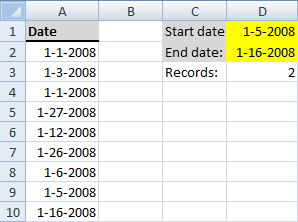Author: Oscar Cronquist Article last updated on November 17, 2018

How do I automatically count dates in a specific date range?Array formula in cell D3:

=SUM(IF((\$A\$2:\$A\$10<\$D\$2)*(\$A\$2:\$A\$10>\$D\$1), 1, 0)) + Ctrl + Shift + Enter

This is an array formula so make sure you press Ctrl + Shift + Enter.

Alternative array formula in cell D3:

=SUMPRODUCT(--(\$A\$2:\$A\$10<\$D\$2),--(\$A\$2:\$A\$10>\$D\$1)) + ENTER

If you want to count the start date and end date also, try this formula:

=SUM(IF((\$A\$2:\$A\$10<=\$D\$2)*(\$A\$2:\$A\$10>=\$D\$1),1,0)) + Ctrl + Shift + Enter

Alternative formula:

=SUMPRODUCT(--(\$A\$2:\$A\$10<=\$D\$2),--(\$A\$2:\$A\$10>=\$D\$1)) + ENTER

### Explaining alternative formula in cell D3

=SUMPRODUCT(--(\$A\$2:\$A\$10<=\$D\$2),--(\$A\$2:\$A\$10>=\$D\$1))

Step 1 - Create a boolean array with matching dates to the first criterion

=SUMPRODUCT(--(\$A\$2:\$A\$10<=\$D\$2),--(\$A\$2:\$A\$10>=\$D\$1))

--(\$A\$2:\$A\$10<=\$D\$2)

becomes

--({39448;39450;39448;39474;39459;39473;39453;39452;39463}<=39463)

becomes

--({39448;39450;39448;39474;39459;39473;39453;39452;39463}<=39463)

becomes

--({TRUE;TRUE;TRUE;FALSE;TRUE;FALSE;TRUE;TRUE;TRUE})

becomes

{1;1;1;0;1;0;1;1;1}

Step 2 - Create a boolean array with matching dates to the second criterion

=SUMPRODUCT(--(\$A\$2:\$A\$10<=\$D\$2),--(\$A\$2:\$A\$10>=\$D\$1))

--(\$A\$2:\$A\$10>=\$D\$1)

becomes

--({39448;39450;39448;39474;39459;39473;39453;39452;39463}>=39452)

becomes

--({FALSE;FALSE;FALSE;TRUE;TRUE;TRUE;TRUE;TRUE;TRUE})

becomes

{0;0;0;1;1;1;1;1;1}

Step 3 - All together

=SUMPRODUCT(--(\$A\$2:\$A\$10<=\$D\$2),--(\$A\$2:\$A\$10>=\$D\$1))

becomes

=SUMPRODUCT({1;1;1;0;1;0;1;1;1},{0;0;0;1;1;1;1;1;1})

becomes

=SUMPRODUCT({0;0;0;0;1;0;1;1;1}) returns 4.